# Early Elementary Math

Sale ends in
Teacher: Caitlin
Customers Who Have Viewed This Course: 1343
\$315.00
\$148.00

#### 0 Course Introduction 03:03

This is the course introduction!  Find out more about what to expect from Early Elementary Math.  This video will explain more specific details about the course and what families can expect.

#### 1 Lesson 1 - Counting a Set 09:45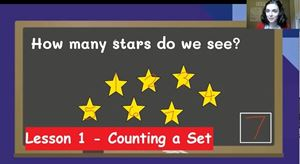Lesson 1 - Counting a Set, focuses on the student's ability to count items in a set and write numbers.  It reviews counting order and introduces a ten frame.  It also allows students to practice counting a set that is spread out instead of arranged in a line.  The lesson has one exercise document for students to complete and 1 exercise review video for students to watch to review the answers and ways to solve the tasks on the exercise.

The lesson video is 9:46.

The exercise contains 5 tasks and should take about 6 minutes to complete.

This lesson most closely aligns with Kindergarten math standard, CCSS.MATH.CONTENT.K.CC.B.5. "Count to answer 'how many?' questions about as many as 20 things arranged in a line, a rectangular array, or a circle, or as many as 10 things in a scattered configuration; given a number from 1-20, count out that many objects."

#### 2 Lesson 1 - Exercise Review 03:34

This video walks students through the answers to the exercise for lesson 1.  The video highlights steps and strategies that students should have used when completing the exercise.

The length of the video is 3:35.

#### 3 Lesson 2 - Teens 14:07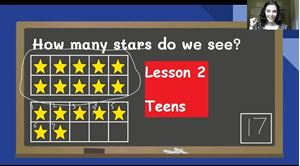Lesson 2 - Teens, focuses on the concept of teens having a full bundle of ten and some ones.  It reviews counting order and ten frames.  It also allows students to practice counting a set within multiple ten frames.  The lesson has one exercise document for students to complete and 1 exercise review video for students to watch to review the answers and ways to solve the tasks on the exercise.

The lesson video is 14:08.

This lesson most closely aligns with First Grade math standard, CCSS.MATH.CONTENT.1.NBT.B.2.B “The numbers from 11 to 19 are composed of a ten and one, two, three, four, five, six, seven, eight, or nine ones.”

#### 4 Lesson 2 - Exercise Review 06:50

This video walks students through the answers to the exercise for lesson 2. The video highlights steps and strategies that students should have used when completing the exercise.

The length of the video is 6:52.

#### 5 Lesson 3 - Patterns Counting to 100 22:27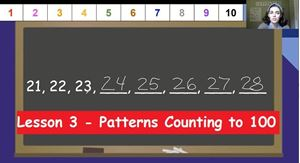Lesson 3 - Patterns Counting to 100, focuses on the patterns of digits when counting to 100.  It also allows students to practice counting on from a given number.  The lesson has one exercise document for students to complete and 1 exercise review video for students to watch to review the answers and ways to solve the tasks on the exercise.

The lesson video is 22:29.

The exercise contains 10 missing numbers in the 100 chart and 3 counting on tasks.  It should take about 15 minutes to complete.

This lesson most closely aligns with First Grade math standard, CSS.MATH.CONTENT.1.NBT.A.1 “Count to 120, starting at any number less than 120. In this range, read and write numerals and represent a number of objects with a written numeral.”

It also aligns with kindergarten standard, CCSS.MATH.CONTENT.K.CC.A.1 "Count to 100 by ones and by tens."

#### 6 Lesson 3 - Exercise Review 09:34

This video walks students through the answers to the exercise for lesson 3. The video highlights steps and strategies that students should have used when completing the exercise.

The length of the video is 9:35.

#### 7 Lesson 4 - Patterns Counting to 150 22:49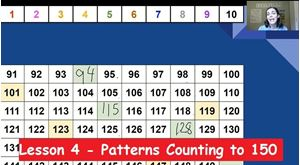Lesson 4- Patterns Counting to 150, focuses on the patterns of digits when counting to 150, especially on the numbers that come after 100.  It also allows students to practice counting on from a given number.  The lesson has one exercise document for students to complete and 1 exercise review video for students to watch to review the answers and ways to solve the tasks on the exercise.

The lesson video is 22:51.

The exercise has 10 missing numbers in a 150 chart and 3 counting on tasks.  It should take about 15 minutes to complete.

This lesson most closely aligns with First Grade math standard, CSS.MATH.CONTENT.1.NBT.A.1 “Count to 120, starting at any number less than 120. In this range, read and write numerals and represent a number of objects with a written numeral.”

Also, this lesson goes up to 150 to more closely align with the NC Standard Course of Study for 1st Grade.

#### 8 Lesson 4 - Exercise Review 08:25

This video walks students through the answers to the exercise for lesson 4. The video highlights steps and strategies that students should have used when completing the exercise.

The length of the video is 8:25.

#### 9 Lesson 5 - Odd and Even 11:28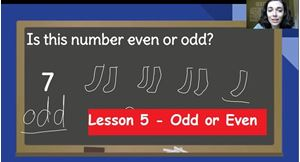Lesson 5 - Odd or Even, focuses on determining if numbers are odd or even.  It shows how to draw pairs to determine if odd or even and it also shows the pattern students can use to know if a number is odd or even.  The lesson has one exercise document for students to complete and 1 exercise review video for students to watch to review the answers and ways to solve the tasks on the exercise.

The lesson video is 11:30.

The exercise contains 8 tasks and should take about 10 minutes to complete.

This lesson most closely aligns with Second Grade math standard, CCSS.MATH.CONTENT.2.OA.C.3 “Determine whether a group of objects (up to 20) has an odd or even number of members, e.g., by pairing objects or counting them by 2s; write an equation to express an even number as a sum of two equal addends.”

#### 10 Lesson 5 - Exercise Review & Quiz 05:14

This video walks students through the answers to the exercise for lesson 5. The video highlights steps and strategies that students should have used when completing the exercise.

The length of the video is 5:14.

After watching the video, please complete the counting quiz. This quiz covers all counting skills that have been taught so far.

#### 11 Lesson 6 - Basic Adding: Objects 13:36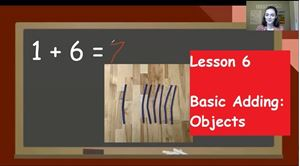Lesson 6 - Basic Adding: Object, focuses on how to use objects to recreate and solve a basic addition equation within 10.  It introduces important addition terminology such as, addend, sum, part, and whole.  The lesson has one exercise document for students to complete and 1 exercise review video for students to watch to review the answers and ways to solve the tasks on the exercise.

The lesson video is 13:38.

The exercise has 8 problems and should take about 10 minutes to complete.

This lesson most closely aligns with Kindergarten math standard, CCSS.MATH.CONTENT.K.OA.A.1 “Represent addition and subtraction with objects, fingers, mental images, drawings, sounds (e.g., claps), acting out situations, verbal explanations, expressions, or equations.”

It also aligns with a First Grade math standard, CCSS.MATH.CONTENT.1.OA.C.6 “Add and subtract within 20, demonstrating fluency for addition and subtraction within 10. Use strategies such as counting on; making ten; decomposing a number leading to a ten; using the relationship between addition and subtraction ; and creating equivalent but easier or known sums.”

#### 12 Lesson 6 - Exercise Review 04:41

This video walks students through the answers to the exercise for lesson 6. The video highlights steps and strategies that students should have used when completing the exercise.

The length of the video is 4:41.

#### 13 Lesson 7 - Basic Adding: Pictures 10:08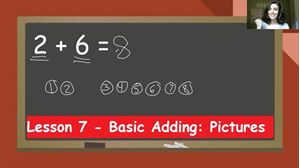Lesson 7- Basic Adding: Picture, focuses on how to draw quick pictures to show and solve a basic addition equation within 10.  It reviews important addition terminology such as, addend, sum, part, and whole.  The lesson has one exercise document for students to complete and 1 exercise review video for students to watch to review the answers and ways to solve the tasks on the exercise.

The lesson video is 10:10.

The exercise has 8 problems and should take about 10 minutes to complete.

This lesson most closely aligns with Kindergarten math standard, CCSS.MATH.CONTENT.K.OA.A.1 “Represent addition and subtraction with objects, fingers, mental images, drawings, sounds (e.g., claps), acting out situations, verbal explanations, expressions, or equations.”

It also aligns with a First Grade math standard, CCSS.MATH.CONTENT.1.OA.C.6 “Add and subtract within 20, demonstrating fluency for addition and subtraction within 10. Use strategies such as counting on; making ten; decomposing a number leading to a ten; using the relationship between addition and subtraction ; and creating equivalent but easier or known sums.”

#### 14 Lesson 7 - Exercise Review 07:34

This video walks students through the answers to the exercise for lesson 7. The video highlights steps and strategies that students should have used when completing the exercise.

The length of the video is 7:36.

#### 15 Lesson 8 - Basic Adding: Counting On 10:33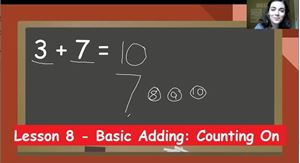Lesson 8- Basic Adding: Counting On, focuses on how to count on with a quick picture to solve a basic addition equation within 10.  It reviews important addition terminology such as, addend, sum, part, and whole.  The lesson has one exercise document for students to complete and 1 exercise review video for students to watch to review the answers and ways to solve the tasks on the exercise.

The lesson video is 10:35.

The exercise has 8 problems on it and should take about 12 minutes to complete.

This lesson most closely aligns with Kindergarten math standard, CCSS.MATH.CONTENT.K.OA.A.1 “Represent addition and subtraction with objects, fingers, mental images, drawings, sounds (e.g., claps), acting out situations, verbal explanations, expressions, or equations.”

It also aligns with a First Grade math standard, CCSS.MATH.CONTENT.1.OA.C.5 “Relate counting to addition and subtraction.”

#### 16 Lesson 8 - Exercise Review 07:59

This video walks students through the answers to the exercise for lesson 8. The video highlights steps and strategies that students should have used when completing the exercise.

The length of the video is 7:59.

#### 17 Lesson 9 - Basic Adding: Number Line 14:40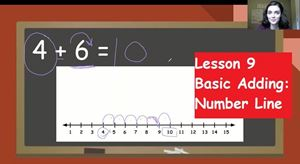Lesson 9 - Basic Adding: Number Line, focuses on how to count on with a number line to solve a basic addition equation within 10.  It reviews important addition terminology such as, addend, sum, part, and whole.  The lesson has one exercise document for students to complete and 1 exercise review video for students to watch to review the answers and ways to solve the tasks on the exercise.

Please print a blank set of number lines to 15 prior to your student watching the lesson video so they can have number lines to do work on during the video lesson.

The lesson video is 14:41.

The exercise has 8 problems and should take about 12 minutes to complete.

This lesson most closely aligns with Kindergarten math standard, CCSS.MATH.CONTENT.K.OA.A.1 “Represent addition and subtraction with objects, fingers, mental images, drawings, sounds (e.g., claps), acting out situations, verbal explanations, expressions, or equations.”

It also aligns with a First Grade math standard, CCSS.MATH.CONTENT.1.OA.C.5 “Relate counting to addition and subtraction.”

#### 18 Lesson 9 - Exercise Review 06:22

This video walks students through the answers to the exercise for lesson 9. The video highlights steps and strategies that students should have used when completing the exercise.

The length of the video is 6:22.

#### 19 Lesson 10 - Basic Subtracting: Objects 12:22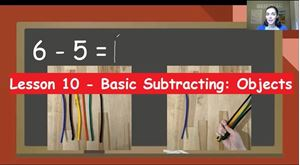Lesson 10 - Basic Subtracting: Objects, focuses on how to use objects to recreate and solve a basic subtraction equation within 10.  It introduces important subtraction terminology such as, minuend, subtrahend, difference, part, and whole.  The lesson has one exercise document for students to complete and 1 exercise review video for students to watch to review the answers and ways to solve the tasks on the exercise.

The lesson video is 12:24.

The exercise has 8 problems and should take about 10 minutes to complete.

This lesson most closely aligns with Kindergarten math standard, CCSS.MATH.CONTENT.K.OA.A.1 “Represent addition and subtraction with objects, fingers, mental images, drawings, sounds (e.g., claps), acting out situations, verbal explanations, expressions, or equations.”

It also aligns with a First Grade math standard, CCSS.MATH.CONTENT.1.OA.C.6 “Add and subtract within 20, demonstrating fluency for addition and subtraction within 10. Use strategies such as counting on; making ten; decomposing a number leading to a ten; using the relationship between addition and subtraction; and creating equivalent but easier or known sums.”

#### 20 Lesson 10 - Exercise Review 06:02

This video walks students through the answers to the exercise for lesson 10. The video highlights steps and strategies that students should have used when completing the exercise.

The length of the video is 6:02.

#### 21 Lesson 11 - Basic Subtracting: Pictures 09:50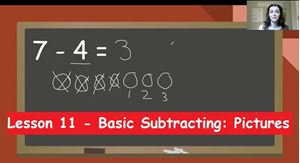Lesson 11 - Basic Subtracting: Pictures, focuses on how to draw quick pictures to represent and solve a basic subtraction equation within 10.  It reviews important subtraction terminology such as, minuend, subtrahend, difference, part, and whole.  The lesson has one exercise document for students to complete and 1 exercise review video for students to watch to review the answers and ways to solve the tasks on the exercise.

The lesson video is 9:52.

The exercise has 8 problems and should take about 10 minutes to complete.

This lesson most closely aligns with Kindergarten math standard, CCSS.MATH.CONTENT.K.OA.A.1 “Represent addition and subtraction with objects, fingers, mental images, drawings, sounds (e.g., claps), acting out situations, verbal explanations, expressions, or equations.”

It also aligns with a First Grade math standard, CCSS.MATH.CONTENT.1.OA.C.6 “Add and subtract within 20, demonstrating fluency for addition and subtraction within 10. Use strategies such as counting on; making ten; decomposing a number leading to a ten; using the relationship between addition and subtraction; and creating equivalent but easier or known sums.”

#### 22 Lesson 11 - Exercise Review 05:16

This video walks students through the answers to the exercise for lesson 11. The video highlights steps and strategies that students should have used when completing the exercise.

The length of the video is 5:16.

#### 23 Lesson 12 - Basic Subtracting: Counting Back 10:09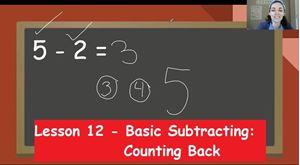Lesson 12 - Basic Subtracting: Counting Back, focuses on how to draw a quick picture to count backward and solve a basic subtraction equation within 10.  It reviews important subtraction terminology such as, minuend, subtrahend, difference, part, and whole.  The lesson has one exercise document for students to complete and 1 exercise review video for students to watch to review the answers and ways to solve the tasks on the exercise.

The lesson video is 10:10.

The exercise has 8 problems and should take about 12 minutes to complete.

This lesson most closely aligns with Kindergarten math standard, CCSS.MATH.CONTENT.K.OA.A.1 “Represent addition and subtraction with objects, fingers, mental images, drawings, sounds (e.g., claps), acting out situations, verbal explanations, expressions, or equations.”

CCSS.MATH.CONTENT.1.OA.C.5 “Relate counting to addition and subtraction.”

#### 24 Lesson 12 - Exercise Review 06:45

This video walks students through the answers to the exercise for lesson 12. The video highlights steps and strategies that students should have used when completing the exercise.

The length of the video is 6:45.

#### 25 Lesson 13 - Basic Subtracting: Number Line 09:34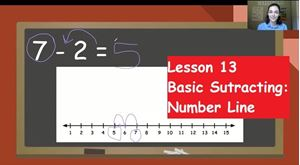Lesson 13 - Basic Subtracting: Number Line, focuses on how to use a number line to count backward and solve a basic subtraction equation within 10.  It reviews important subtraction terminology such as, minuend, subtrahend, difference, part, and whole.  The lesson has one exercise document for students to complete and 1 exercise review video for students to watch to review the answers and ways to solve the tasks on the exercise.

Please print a blank set of number lines to 15 prior to your student watching the lesson video so they can have number lines to do work on during the video lesson.

The lesson video is 9:35.

The exercise has 8 problems and should take about 12 minutes to complete.

This lesson most closely aligns with Kindergarten math standard, CCSS.MATH.CONTENT.K.OA.A.1 “Represent addition and subtraction with objects, fingers, mental images, drawings, sounds (e.g., claps), acting out situations, verbal explanations, expressions, or equations.”

CCSS.MATH.CONTENT.1.OA.C.5 “Relate counting to addition and subtraction.”

#### 26 Lesson 13 - Exercise Review & Quiz 05:57

This video walks students through the answers to the exercise for lesson 13. The video highlights steps and strategies that students should have used when completing the exercise.

The length of the video is 5:57.

After watching the video please complete the Addition and Subtraction Quiz. This quiz covers basic addition and subtraction that has been taught so far.

#### 27 Lesson 14 - Story Problems: Addition 14:24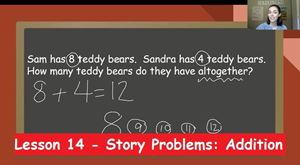Lesson 14 - Story Problems: Addition, focuses on how to create an addition equation base on a story problem and how to solve for the answer.  It reviews important addition terminology such as, addend, sum, part, and whole.  The lesson has one exercise document for students to complete and 1 exercise review video for students to watch to review the answers and ways to solve the tasks on the exercise.

The lesson video is 14:25.

The exercise has 4 problems and should take about 12 minutes to complete.

This lesson most closely aligns with First Grade math standard, CCSS.MATH.CONTENT.1.OA.A.1 “Use addition and subtraction within 20 to solve word problems involving situations of adding to, taking from, putting together, taking apart, and comparing, with unknowns in all positions, e.g., by using objects, drawings, and equations with a symbol for the unknown number to represent the problem.”

This lesson is also related to the following standards.

CCSS.MATH.CONTENT.K.OA.A.2 “Solve addition and subtraction word problems, and add and subtract within 10, e.g., by using objects or drawings to represent the problem.”

#### 28 Lesson 14 - Exercise Review 06:05

This video walks students through the answers to the exercise for lesson 14. The video highlights steps and strategies that students should have used when completing the exercise.

The length of the video is 6:05.

#### 29 Lesson 15 - Story Problems: Subtraction 11:32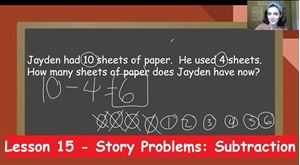Lesson 15 - Story Problems: Subtraction, focuses on how to create a subtraction equation based on a story problem and how to solve for the answer.  It reviews important subtraction terminology such as, minuend, subtrahend, difference, part, and whole.  The lesson has one exercise document for students to complete and 1 exercise review video for students to watch to review the answers and ways to solve the tasks on the exercise.

The lesson video is 11:33.

The exercise contains 4 problems and should take about 12 minutes to complete.

This lesson most closely aligns with First Grade math standard, CCSS.MATH.CONTENT.1.OA.A.1 “Use addition and subtraction within 20 to solve word problems involving situations of adding to, taking from, putting together, taking apart, and comparing, with unknowns in all positions, e.g., by using objects, drawings, and equations with a symbol for the unknown number to represent the problem.”

This lesson is also related to the following standards.

CCSS.MATH.CONTENT.K.OA.A.2 “Solve addition and subtraction word problems, and add and subtract within 10, e.g., by using objects or drawings to represent the problem.”

#### 30 Lesson 15 - Exercise Review 06:24

This video walks students through the answers to the exercise for lesson 15. The video highlights steps and strategies that students should have used when completing the exercise.

The length of the video is 6:24.

#### 31 Lesson 16 - Story Problems: Addition and Subtraction 13:42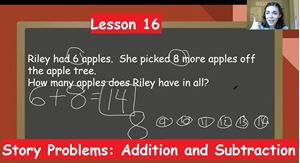Lesson 16 - Story Problems: Addition and Subtraction, focuses on how to determine the operation when creating an equation based on a story problem and how to solve for the answer.  This lesson introduces the term operation and reviews important terminology such as, addend, sum, minuend, subtrahend, difference, part, and whole.  The lesson has one exercise document for students to complete and 1 exercise review video for students to watch to review the answers and ways to solve the tasks on the exercise.

The lesson video is 13:44.

The exercise contains 4 questions and should take about 12 minutes.

This lesson most closely aligns with First Grade math standard, CCSS.MATH.CONTENT.1.OA.A.1 “Use addition and subtraction within 20 to solve word problems involving situations of adding to, taking from, putting together, taking apart, and comparing, with unknowns in all positions, e.g., by using objects, drawings, and equations with a symbol for the unknown number to represent the problem.”

This lesson is also related to the following standards.

CCSS.MATH.CONTENT.K.OA.A.2 “Solve addition and subtraction word problems, and add and subtract within 10, e.g., by using objects or drawings to represent the problem.”

#### 32 Lesson 16 - Exercise Review & Quiz 06:36

This video walks students through the answers to the exercise for lesson 16. The video highlights steps and strategies that students should have used when completing the exercise.

The length of the video is 6:36.

After the video, please complete the Story Problems Quiz. This quiz covers basic addition and subtraction story problems.

#### 33 Lesson 17 - True or False Math 19:37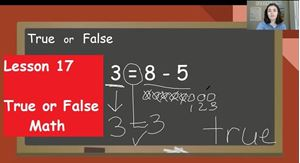Lesson 17 - True or False Math, focuses on how to determine if equations written are true or false.  This lesson reviews the meaning of the equal sign in an equation.  This lesson introduces the terms true and false.  To complete this activity, students must be able to accurately solve basic addition and subtraction problems independently.  The lesson has one exercise document for students to complete and 1 exercise review video for students to watch to review the answers and ways to solve the tasks on the exercise.

The lesson video is 19:39.

The exercise contains 8 problems and should take about 20 minutes.

This lesson most closely aligns with First Grade math standard, CCSS.MATH.CONTENT.1.OA.D.7 “Understand the meaning of the equal sign, and determine if equations involving addition and subtraction are true or false.”

#### 34 Lesson 17 - Exercise Review 12:10

This video walks students through the answers to the exercise for lesson 17. The video highlights steps and strategies that students should have used when completing the exercise.

The length of the video is 12:12

#### 35 Lesson 18 - Unknown Parts 22:12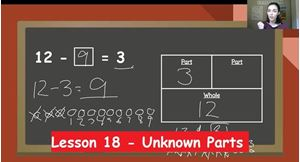Lesson 18 - Unknown Parts, focuses on how to solve unknown parts problems.  This lesson reviews the parts and the whole in addition and subtraction equations.  This lesson teaches how to turn an unknown part equation into a solvable subtraction equation.  To complete this activity, students must be able to accurately solve basic subtraction problems independently.  The lesson has two exercise documents for students to complete and 2 exercise review videos for students to watch to review the answers and ways to solve the tasks on the exercise.

The lesson video is 22:13.

Exercise 1 contains 6 problems and should take about 15 minutes.

Exercise 2 contains 6 problems and should take about 15 minutes.

Please make sure that the student watches the matching exercise review video shortly after finishing the exercise.

This lesson most closely aligns with First Grade math standard, CCSS.MATH.CONTENT.1.OA.D.8 “Determine the unknown whole number in an addition or subtraction equation relating three whole numbers.”

#### 36 Lesson 18 - Exercise 1 Review 10:22

This video walks students through the answers to the exercise 1 for lesson 18. The video highlights steps and strategies that students should have used when completing the exercise.

The length of the video is 10:22.

#### 37 Lesson 18 - Exercise 2 Review 08:53

This video walks students through the answers to the exercise 2 for lesson 18. The video highlights steps and strategies that students should have used when completing the exercise.

The length of the video is 8:55.

#### 38 Lesson 19 - Unknown Part Story Problems 18:55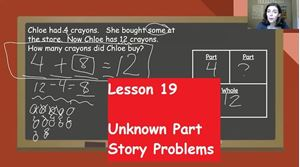Lesson 19 - Unknown Part Story Problems, focuses on how to turn story problems into unknown parts equations then solve.  This lesson reviews the parts and the whole in addition and subtraction equations.  This lesson reviews how to turn an unknown part equation into a solvable subtraction equation.  To complete this activity, students must be able to accurately determine the operation in a story problem.  The lesson has two exercise documents for students to complete and 2 exercise review videos for students to watch to review the answers and ways to solve the tasks on the exercise.

The lesson video is 18:57.

Exercise 1 contains 4 problems and should take about 15 minutes.

Exercise 2 contains 4 problems and should take about 15 minutes.

Please make sure that the student watches the matching exercise review video shortly after finishing the exercise and before moving on.

This lesson most closely aligns with First Grade math standards, CCSS.MATH.CONTENT.1.OA.D.8 “Determine the unknown whole number in an addition or subtraction equation relating three whole numbers.”

and

CCSS.MATH.CONTENT.1.OA.A.1 “Use addition and subtraction within 20 to solve word problems involving situations of adding to, taking from, putting together, taking apart, and comparing, with unknowns in all positions, e.g., by using objects, drawings, and equations with a symbol for the unknown number to represent the problem.”

#### 39 Lesson 19 - Exercise 1 Review 10:33

This video walks students through the answers to the exercise 1 for lesson 19. The video highlights steps and strategies that students should have used when completing the exercise.

The length of the video is 10:33.

#### 40 Lesson 19 - Exercise 2 Review & Quiz 11:13

This video walks students through the answers to the exercise 2 for lesson 19. The video highlights steps and strategies that students should have used when completing the exercise.

The length of the video is 11:13.

After the video, please complete the True or False and Unknown Parts Quiz.

#### 41 Lesson 20 - Three Addends 18:49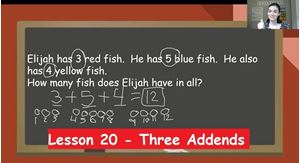Lesson 20 - Three Addends, focuses on how to turn story problems into addition equations then solve.  This lesson reviews addition terminology addend and sum.  This lesson introduces putting 3 addends together.  To complete this activity, students must be able to accurately solve basic addition problems.  The lesson has one exercise document for students to complete and 1 exercise review video for students to watch to review the answers and ways to solve the tasks on the exercise.

The lesson video is 18:51.

The exercise contains 4 problems and should take about 10 minutes.

Please make sure that the student watches the matching exercise review video shortly after finishing the exercise and before moving on.

This lesson most closely aligns with First Grade math standard, CCSS.MATH.CONTENT.1.OA.A.2 “Solve word problems that call for addition of three whole numbers whose sum is less than or equal to 20, e.g., by using objects, drawings, and equations with a symbol for the unknown number to represent the problem.”

#### 42 Lesson 20 - Exercise Review 05:51

This video walks students through the answers to the exercise for lesson 20. The video highlights steps and strategies that students should have used when completing the exercise.

The length of the video is 5:51.

#### 43 Lesson 21 - Properties of Addition 21:33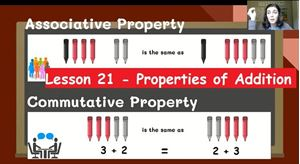Lesson 21 - Properties of Addition, focuses on the commutative and associative properties of addition.  This lesson introduces addition terminology commutative property and associative.  This lesson is focused on finding different equations that will have the same sum due to the properties.  The lesson has one exercise document for students to complete and 1 exercise review video for students to watch to review the answers and ways to solve the tasks on the exercise.

The lesson video is 21:35.

The exercise contains 2 matching sets and should take about 10 minutes.

Please make sure that the student watches the matching exercise review video shortly after finishing the exercise and before moving on.

This lesson most closely aligns with First Grade math standard, CCSS.MATH.CONTENT.1.OA.B.3 “Apply properties of operations as strategies to add and subtract. Examples: If 8 + 3 = 11 is known, then 3 + 8 = 11 is also known. (Commutative property of addition.) To add 2 + 6 + 4, the second two numbers can be added to make a ten, so 2 + 6 + 4 = 2 + 10 = 12. (Associative property of addition.)”

#### 44 Lesson 21 - Exercise Review & TEST 07:10

This video walks students through the answers to the exercise for lesson 21. The video highlights steps and strategies that students should have used when completing the exercise.

The length of the video is 7:10.

After watching the video, please complete the Counting and Operations Test.  This test covers all math topics we have learned so far.

#### 45 Lesson 22 - 2D Shapes 17:24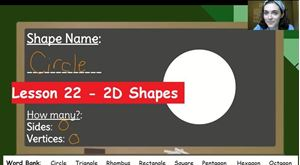Lesson 22 - 2D Shapes, naming and describing the attributes of 2D shapes.  This lesson introduces terms such as sides and vertices, along with the names of shapes circle, triangle, rhombus, rectangle, square, pentagon, hexagon, and octagon.  The lesson has one exercise document for students to complete and 1 exercise review video for students to watch to review the answers and ways to solve the tasks on the exercise.

The lesson video is 17:26.

The exercise contains 8 shapes to name and describe it should take about 12 minutes to complete.

Please make sure that the student watches the matching exercise review video shortly after finishing the exercise and before moving on.

This lesson most closely aligns with First Grade math standard, CCSS.MATH.CONTENT.1.G.A.1 “Distinguish between defining attributes (e.g., triangles are closed and three-sided) versus non-defining attributes (e.g., color, orientation, overall size); build and draw shapes to possess defining attributes.”

This lesson is also related to the following standards.

Second Grade: CCSS.MATH.CONTENT.2.G.A.1 “Recognize and draw shapes having specified attributes, such as a given number of angles or a given number of equal faces.1 Identify triangles, quadrilaterals, pentagons, hexagons, and cubes.”

and

Kindergarten: CCSS.MATH.CONTENT.K.G.A.2 “Correctly name shapes regardless of their orientations or overall size.”

#### 46 Lesson 22 - Exercise Review 05:59

This video walks students through the answers to the exercise for lesson 22. The video highlights steps and strategies that students should have used when completing the exercise.

The length of the video is 5:59.

#### 47 Lesson 23 - Composite Shapes 12:56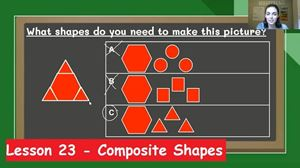Lesson 23 - Composite Shapes, focuses on recognizing and naming 2D shapes that are part of a larger composite shape.  This lesson introduces the term composite and reviews previously taught shape vocabulary.  To complete this activity students need to be able to correctly identify 2D shapes.  The lesson has one exercise document for students to complete and 1 exercise review video for students to watch to review the answers and ways to solve the tasks on the exercise.

The lesson video is 12:58.

The exercise contains 4 tasks it should take about 10 minutes to complete.

Please make sure that the student watches the matching exercise review video shortly after finishing the exercise and before moving on.

This lesson most closely aligns with First Grade math standard, CCSS.MATH.CONTENT.1.G.A.2 “Compose two-dimensional shapes (rectangles, squares, trapezoids, triangles, half-circles, and quarter-circles) or three-dimensional shapes (cubes, right rectangular prisms, right circular cones, and right circular cylinders) to create a composite shape, and compose new shapes from the composite shape.”

#### 48 Lesson 23 - Exercise Review 05:35

This video walks students through the answers to the exercise for lesson 23. The video highlights steps and strategies that students should have used when completing the exercise.

The length of the video is 5:35.

#### 49 Lesson 24 - 3D Shapes 28:54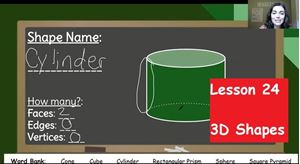Lesson 24 - 3D Shapes, focuses on recognizing and naming 3D shapes.  This lesson introduces the terms edges and faces, while introducing sphere, cone, cylinder, square pyramid, cube, and rectangular prism.  This lesson also reviews previously taught shape vocabulary.  To complete this activity students need to be able to correctly identify 2D shapes.  The lesson has one exercise document for students to complete and 1 exercise review video for students to watch to review the answers and ways to solve the tasks on the exercise.

The lesson video is 28:56.

The exercise contains 6 shapes to name and describe it should take about 10 minutes to complete.

Please make sure that the student watches the matching exercise review video shortly after finishing the exercise and before moving on.

This lesson most closely aligns with First Grade math standard, CCSS.MATH.CONTENT.1.G.A.1 “Distinguish between defining attributes (e.g., triangles are closed and three-sided) versus non-defining attributes (e.g., color, orientation, overall size); build and draw shapes to possess defining attributes.”

This lesson is also related to the following standard.

Second Grade: CCSS.MATH.CONTENT.2.G.A.1 “Recognize and draw shapes having specified attributes, such as a given number of angles or a given number of equal faces.1 Identify triangles, quadrilaterals, pentagons, hexagons, and cubes.”

and

Kindergarten: CCSS.MATH.CONTENT.K.G.A.2 “Correctly name shapes regardless of their orientations or overall size.”

#### 50 Lesson 24 - Exercise Review 07:04

This video walks students through the answers to the exercise for lesson 24. The video highlights steps and strategies that students should have used when completing the exercise.

The length of the video is 7:04.

#### 51 Lesson 25 - 2D vs. 3D Shapes 14:48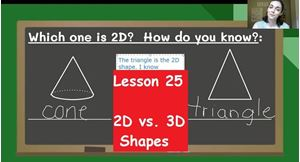Lesson 25 - 2D vs. 3D Shapes, focuses on differentiating between 2D and 3D shapes.  This lesson focuses on the clues that help students tell if a shape is solid or flat. This lesson reviews the attributes and shape names previously taught.  To complete this activity students need to be able to correctly identify 2D shapes and 3D shapes.  The lesson has one exercise document for students to complete and 1 exercise review video for students to watch to review the answers and ways to solve the tasks on the exercise.

The lesson video is 14:50.

The exercise contains a sorting activity and 6 shapes to name.  It should take about 10 minutes to complete.

Please make sure that the student watches the matching exercise review video shortly after finishing the exercise and before moving on.

This lesson most closely aligns with Kindergarten math standards, CCSS.MATH.CONTENT.K.G.A.3 “Identify shapes as two-dimensional (lying in a plane, "flat") or three-dimensional ("solid").”

and

CCSS.MATH.CONTENT.K.G.B.4 “Analyze and compare two- and three-dimensional shapes, in different sizes and orientations, using informal language to describe their similarities, differences, parts (e.g., number of sides and vertices/"corners") and other attributes (e.g., having sides of equal length).”

This lesson is also related to the following standards.

Second Grade: CCSS.MATH.CONTENT.2.G.A.1 “Recognize and draw shapes having specified attributes, such as a given number of angles or a given number of equal faces.1 Identify triangles, quadrilaterals, pentagons, hexagons, and cubes.”

and

Kindergarten: CCSS.MATH.CONTENT.K.G.A.2 “Correctly name shapes regardless of their orientations or overall size.”

#### 52 Lesson 25 - Exercise Review & Quiz 08:13

This video walks students through the answers to the exercise for lesson 25. The video highlights steps and strategies that students should have used when completing the exercise.

The length of the video is 8:15.

After the video, print the document named SHAPES QUIZ PICTURES. Then make sure the student has that document next to them as they take the quiz.  This Shapes Quiz will cover the names and attributes of some shapes that have been taught.

#### 53 Lesson 26 - Fractions 23:18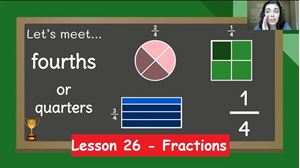Lesson 26 - Fractions, focuses on identifying fractions by the number of pieces.  This lesson also addresses the requirements to be considered real fractions. This lesson also allows student to draw lines to partition their own shapes into halves, thirds, and fourths.  New terminology is introduced such as, whole, half, third, fourth and quarter. The lesson has two exercise documents for students to complete and 2 exercise review videos for students to watch to review the answers and ways to solve the tasks on the exercise.

The lesson video is 23:20.

Exercise 1 contains a sorting activity, 4 questions about naming and 2 shapes to partition.  It should take about 12 minutes to complete.

Exercise 2 contains 4 questions about naming and 4 shapes to partition.  It should take about 10 minutes to complete.

Please make sure that the student watches the matching exercise review video shortly after finishing the exercise and before moving on.

This lesson most closely aligns with First Grade math standards, CCSS.MATH.CONTENT.1.G.A.3 “Partition circles and rectangles into two and four equal shares, describe the shares using the words halves, fourths, and quarters, and use the phrases half of, fourth of, and quarter of. Describe the whole as two of, or four of the shares. Understand for these examples that decomposing into more equal shares creates smaller shares.”

This lesson is also related to the following standards.

Second Grade: CCSS.MATH.CONTENT.2.G.A.3 “Partition circles and rectangles into two, three, or four equal shares, describe the shares using the words halves, thirds, half of, a third of, etc., and describe the whole as two halves, three thirds, four fourths. Recognize that equal shares of identical wholes need not have the same shape.”

#### 54 Lesson 26 - Exercise 1 Review 05:33

This video walks students through the answers to the exercise 1 for lesson 26. The video highlights steps and strategies that students should have used when completing the exercise.

The length of the video is 5:35.

#### 55 Lesson 26 - Exercise 2 Review & TEST 06:48

This video walks students through the answers to the exercise 2 for lesson 26. The video highlights steps and strategies that students should have used when completing the exercise.

The length of the video is 6:48.

After the video, print the document named GEOMETRY TEST PICTURES. Then make sure the student has that document next to them as they take the test.  This Geometry Test will cover the names and attributes of some shapes and fractions that have been taught.  The Geometry Test will also include a few review questions from the first unit.

#### 56 Lesson 27 - Coins 17:12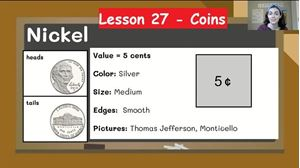Lesson 27 - Coins, focuses on identifying coins and knowing the value of each coin.  This lesson also discusses the visual cues that students can use to differentiate between the 4 coins. This lesson also discusses the value of each coin. New terminology is introduced such as, cents, penny, nickel, dime, and quarter. The lesson has one exercise document for students to complete and 1 exercise review video for students to watch to review the answers and ways to solve the tasks on the exercise.

The lesson video is 17:14.

The exercise contains 8 pictures of coins to identify and 4 questions about value.  It should take about 10 minutes to complete.

Please make sure that the student watches the matching exercise review video shortly after finishing the exercise and before moving on.

This lesson most closely aligns with First Grade math standards from the North Carolina Standard Course of Study, NC.1.MD.5 “Identify quarters, dimes, and nickels and relate their values to pennies.”

This lesson is also related to the following standards.

Second Grade: CCSS.MATH.CONTENT.2.MD.C.8 “Solve word problems involving dollar bills, quarters, dimes, nickels, and pennies, using \$ and ¢ symbols appropriately. Example: If you have 2 dimes and 3 pennies, how many cents do you have?”

#### 57 Lesson 27 - Exercise Review 06:26

This video walks students through the answers to the exercise for lesson 27. The video highlights steps and strategies that students should have used when completing the exercise.

The length of the video is 6:26.

#### 58 Lesson 28 - Counting Coins 17:42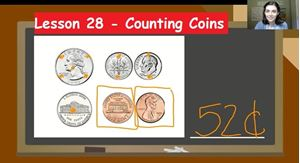Lesson 28 - Counting Coins, focuses on using skip counting by 5 to help count up the value of a group of coins.  This lesson reviews how to distinguish coins from one another. Coin terminology such as, cents, penny, nickel, dime, and quarter, is reviewed in this lesson. The lesson has two exercise documents for students to complete and 2 exercise review videos for students to watch to review the answers and ways to solve the tasks on the exercise.

The lesson video is 17:44.

Exercise 1 contains 8 rows of coins for students to count.  Each row contains only one type of coin.  It should take about 12 minutes to complete.

Exercise 2 contains 7 rows of coins for students to count.  Each row contains multiple types of coins.  It should take about 12 minutes to complete.

Please make sure that the student watches the matching exercise review video shortly after finishing the exercise and before moving on.

This lesson most closely aligns with Second Grade: CCSS.MATH.CONTENT.2.MD.C.8 “Solve word problems involving dollar bills, quarters, dimes, nickels, and pennies, using \$ and ¢ symbols appropriately. Example: If you have 2 dimes and 3 pennies, how many cents do you have?”

This lesson is also related to the following standards.

First Grade math standards from the North Carolina Standard Course of Study, NC.1.MD.5 “Identify quarters, dimes, and nickels and relate their values to pennies.”

#### 59 Lesson 28 - Exercise 1 Review 04:48

This video walks students through the answers to the exercise 1 for lesson 28. The video highlights steps and strategies that students should have used when completing the exercise.

The length of the video is 4:48.

#### 60 Lesson 28 - Exercise 2 Review & Quiz 04:59

This video walks students through the answers to the exercise 2 for lesson 28. The video highlights steps and strategies that students should have used when completing the exercise.

The length of the video is 4:59.

After the video, complete the Coins Quiz.  Be sure that print the COINS QUIZ PICTURES document before students begin the quiz. Students must look the pictures while taking the quiz.  This quiz covers the names of coins and counting up groups of mixed coins.

#### 61 Lesson 29 - Telling Time: to the Hour 18:50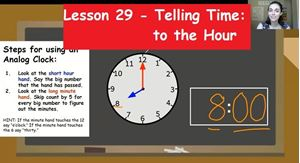Lesson 29 - Telling Time: to the Hour, focuses on introducing terminology hour hand, minute hand, analog clock, digital clock, and colon.  This lesson teaches students how to tell and write time to the hour. The lesson has one exercise document for students to complete and 1 exercise review video for students to watch to review the answers and ways to solve the tasks on the exercise.

The lesson video is 18:52.

The exercise contains a matching section, 4 clocks for telling time, and 2 clocks to draw hands on.  It should take about 12 minutes to complete.

Please make sure that the student watches the matching exercise review video shortly after finishing the exercise and before moving on.

This lesson most closely aligns with First Grade: CCSS.MATH.CONTENT.1.MD.B.3 “Tell and write time in hours and half-hours using analog and digital clocks.”

This lesson is also related to the following standard.

Second Grade: CCSS.MATH.CONTENT.2.MD.C.7 “Tell and write time from analog and digital clocks to the nearest five minutes, using a.m. and p.m.”

#### 62 Lesson 29 - Exercise Review 05:50

This video walks students through the answers to the exercise for lesson 29. The video highlights steps and strategies that students should have used when completing the exercise.

The length of the video is 5:50.

#### 63 Lesson 30 - Telling Time: to the Half Hour 20:18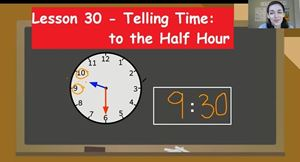Lesson 30 - Telling Time: to the Half Hour, focuses on how to tell and write time to the hour. This lesson reminds students about clock terminology such as, hour hand, minute hand, analog clock, digital clock, and colon.  The lesson has two exercise documents for students to complete and 2 exercise review videos for students to watch to review the answers and ways to solve the tasks on the exercise.

The lesson video is 20:20.

The Time to the Half Hour Exercise contains a matching section, 4 clocks for telling time, and 2 clocks for drawing hands.  It should take about 12 minutes to complete.

The Time to the Hour and Half Hour Exercise contains 6 clocks for telling time and 4 clocks for drawing hands. It should take about 13 minutes to complete.

Please make sure that the student watches the matching exercise review video shortly after finishing the exercise and before moving on.

This lesson most closely aligns with First Grade: CCSS.MATH.CONTENT.1.MD.B.3 “Tell and write time in hours and half-hours using analog and digital clocks.”

This lesson is also related to the following standard.

Second Grade: CCSS.MATH.CONTENT.2.MD.C.7 “Tell and write time from analog and digital clocks to the nearest five minutes, using a.m. and p.m.”

#### 64 Lesson 30 - Time to the Half Hour Exercise Review 07:06

This video walks students through the answers to the Time to the Half Hour Exercise for lesson 30. The video highlights steps and strategies that students should have used when completing the exercise.

The length of the video is 7:08.

#### 65 Lesson 30 - Time to the Hour and Half Hour Exercise Review 06:34

This video walks students through the answers to the Time to the Hour and Half Hour Exercise for lesson 30. The video highlights steps and strategies that students should have used when completing the exercise.

The length of the video is 6:36.

#### 66 Lesson 31 - Telling Time: Increments of 5 18:33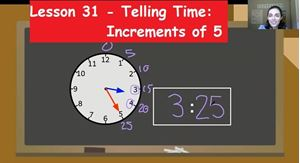Lesson 31 - Telling Time: Increments of 5, focuses on how to tell and write time to the in various 5 minute increments. This lesson reminds students about clock terminology such as, hour hand, minute hand, analog clock, digital clock, and colon.  To complete these exercises, students need to be able to skip count by 5 independently. The lesson has two exercise documents for students to complete and 2 exercise review videos for students to watch to review the answers and ways to solve the tasks on the exercise.

The lesson video is 18:35.

Exercise 1 contains 6 clocks for telling time and 4 clocks for drawing hands. It should take about 15 minutes to complete.

Exercise 2 contains 6 clocks for telling time and 4 clocks for drawing hands. It should take about 15 minutes to complete.

Please make sure that the student watches the matching exercise review video shortly after finishing the exercise and before moving on.

This lesson most closely aligns with Second Grade: CCSS.MATH.CONTENT.2.MD.C.7 “Tell and write time from analog and digital clocks to the nearest five minutes, using a.m. and p.m.”

This lesson is also related to the following standard.

First Grade: CCSS.MATH.CONTENT.1.MD.B.3 “Tell and write time in hours and half-hours using analog and digital clocks.”

#### 67 Lesson 31 - Exercise 1 Review 08:18

This video walks students through the answers to the exercise 1 for lesson 31. The video highlights steps and strategies that students should have used when completing the exercise.

The length of the video is 8:20.

#### 68 Lesson 31 - Exercise 2 Review & Quiz 08:26

This video walks students through the answers to the exercise 2 for lesson 31. The video highlights steps and strategies that students should have used when completing the exercise.

The length of the video is 8:26.

After this video, print out the TELLING TIME QUIZ PICTURES.  Then have students use the pictures during the quiz.  This quiz covers telling time to the hour, half hour, and in increments of 5.

#### 69 Lesson 32 - Comparing Lengths 20:08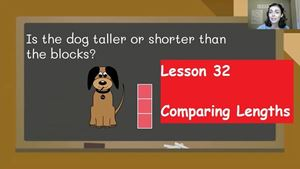Lesson 32 - Comparing Lengths, focuses on deciding which object longest when next to each other or when next to the same item. This lesson reminds students about length terminology such as, taller, shorter, longer, and wider.  The lesson has one exercise document for students to complete and 1 exercise review video for students to watch to review the answers and ways to solve the tasks on the exercise.

The lesson video is 20:10.

The exercise contains 4 tasks to compare lengths directly, 2 tasks to compare lengths indirectly, and 1 story problem to order objects by length. It should take about 10 minutes to complete.

Please make sure that the student watches the matching exercise review video shortly after finishing the exercise and before moving on.

This lesson most closely aligns with First Grade: CCSS.MATH.CONTENT.1.MD.A.1 “Order three objects by length; compare the lengths of two objects indirectly by using a third object.”

#### 70 Lesson 32 - Exercise Review 06:55

This video walks students through the answers to the exercise for lesson 32. The video highlights steps and strategies that students should have used when completing the exercise.

The length of the video is 6:57.

#### 71 Lesson 33 - Measuring with Nonstandard Units 13:55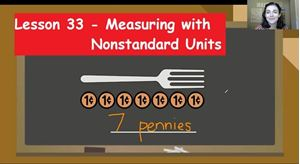Lesson 33 - Measuring with Nonstandard Units, focuses on how to measure objects with nonstandard units. This lesson teaches students to measure end to end, with no gaps, and no overlaps.  The lesson has one exercise document for students to complete and 1 exercise review video for students to watch to review the answers and ways to solve the tasks on the exercise.

The lesson video is 13:57.

The exercise contains 3 tasks to measure and 2 tasks to describe if someone is measuring properly. It should take about 10 minutes to complete.

Please make sure that the student watches the matching exercise review video shortly after finishing the exercise and before moving on.

This lesson most closely aligns with First Grade: CCSS.MATH.CONTENT.1.MD.A.2 “Express the length of an object as a whole number of length units, by laying multiple copies of a shorter object (the length unit) end to end; understand that the length measurement of an object is the number of same-size length units that span it with no gaps or overlaps. Limit to contexts where the object being measured is spanned by a whole number of length units with no gaps or overlaps.”

#### 72 Lesson 33 - Exercise Review 03:32

This video walks students through the answers to the exercise for lesson 33. The video highlights steps and strategies that students should have used when completing the exercise.

The length of the video is 3:32.

#### 73 Lesson 34 - Measuring with a Ruler 11:42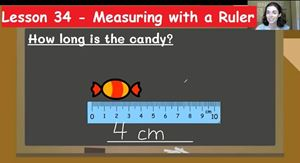Lesson 34 - Measuring with Rulers, focuses on how to measure objects with a ruler. This lesson teaches students to line up the object and ruler properly.  It introduces units inches and centimeters.  The lesson has one exercise document for students to complete and 1 exercise review video for students to watch to review the answers and ways to solve the tasks on the exercise.

The lesson video is 11:44.

The exercise contains 4 tasks to measure and 1 task to choose which picture shows measuring with a ruler properly. It should take about 8 minutes to complete.

Please make sure that the student watches the matching exercise review video shortly after finishing the exercise and before moving on.

This lesson most closely aligns with Second Grade: CCSS.MATH.CONTENT.2.MD.A.1 “Measure the length of an object by selecting and using appropriate tools such as rulers, yardsticks, meter sticks, and measuring tapes.”

#### 74 Lesson 34 - Exercise Review 02:49

This video walks students through the answers to the exercise for lesson 34. The video highlights steps and strategies that students should have used when completing the exercise.

The length of the video is 2:49.

#### 75 Lesson 35 - How Much Longer: Rulers 18:39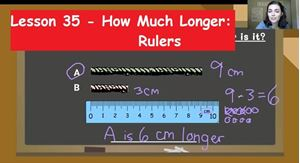Lesson 35 - How Much Longer: Rulers, focuses on measuring 2 items then subtracting to find the difference. This lesson reviews measuring with a ruler.  This lesson builds understanding of subtraction to compare two items.  The lesson has one exercise document for students to complete and 1 exercise review video for students to watch to review the answers and ways to solve the tasks on the exercise.

The lesson video is 18:41.

The exercise contains 6 tasks to compare the lengths of pencils and it should take about 15 minutes to complete.

Please make sure that the student watches the matching exercise review video shortly after finishing the exercise and before moving on.

This lesson most closely aligns with Second Grade: CCSS.MATH.CONTENT.2.MD.A.4 “Measure to determine how much longer one object is than another, expressing the length difference in terms of a standard length unit.”

#### 76 Lesson 35 - Exercise Review 07:30

This video walks students through the answers to the exercise for lesson 35. The video highlights steps and strategies that students should have used when completing the exercise.

The length of the video is 7:30.

#### 77 Lesson 36 - How Much Longer: Story Problems 15:14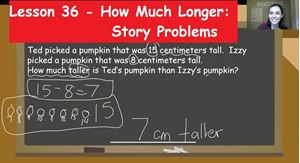Lesson 36 - How Much Longer: Story Problems, focuses on using information from a story problem to create a subtraction equation and then find the difference.  This lesson builds understanding of subtraction to compare two items.  This lesson reviews subtraction terminology. The lesson has one exercise document for students to complete and 1 exercise review video for students to watch to review the answers and ways to solve the tasks on the exercise.

The lesson video is 15:16.

The exercise contains 4 story problems about comparing length and it should take about 12 minutes to complete.

Please make sure that the student watches the matching exercise review video shortly after finishing the exercise and before moving on.

This lesson most closely aligns with First Grade: CCSS.MATH.CONTENT.1.OA.A.1 “Use addition and subtraction within 20 to solve word problems involving situations of adding to, taking from, putting together, taking apart, and comparing, with unknowns in all positions, e.g., by using objects, drawings, and equations with a symbol for the unknown number to represent the problem.”

#### 78 Lesson 36 - Exercise Review & Quiz 06:01

This video walks students through the answers to the exercise for lesson 36. The video highlights steps and strategies that students should have used when completing the exercise.

The length of the video is 6:01.

After watching the video, students should print off the MEASUREMENT QUIZ PICTURES to use while they complete the Measurement Quiz. The Measurement Quiz covers, putting items in order by length, measuring with nonstandard units, measuring with rulers, and determining "how much longer."

#### 79 Lesson 37 - Data: Pictographs 26:52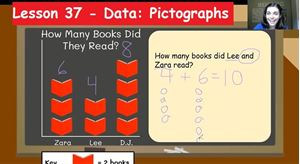Lesson 37 - Data: Pictographs, focuses on answering questions about data shown through a pictograph.  This lesson introduces new terminology data, pictograph, and key. The lesson has two exercise documents for students to complete and 2 exercise review videos for students to watch to review the answers and ways to solve the tasks on the exercise.

The lesson video is 26:53.

Exercise 1 contains data to be drawn into a pictograph and 4 questions to analyze the data. It should take about 12 minutes to complete.

Exercise 2 contains a pictograph and 5 questions to analyze the data. It should take about 10 minutes to complete.

Please make sure that the student watches the matching exercise review video shortly after finishing the exercise and before moving on.

This lesson most closely aligns with First Grade: CCSS.MATH.CONTENT.1.MD.C.4 “Organize, represent, and interpret data with up to three categories; ask and answer questions about the total number of data points, how many in each category, and how many more or less are in one category than in another.”

This lesson is also related to the following standard.

Second Grade: CCSS.MATH.CONTENT.2.MD.D.10 “Draw a picture graph and a bar graph (with single-unit scale) to represent a data set with up to four categories. Solve simple put-together, take-apart, and compare problems using information presented in a bar graph.”

and

Kindergarten: CCSS.MATH.CONTENT.K.MD.B.3 “Classify objects into given categories; count the numbers of objects in each category and sort the categories by count.”

#### 80 Lesson 37 - Exercise 1 Review 06:01

This video walks students through the answers to the exercise 1 for lesson 37. The video highlights steps and strategies that students should have used when completing the exercise.

The length of the video is 6:01.

#### 81 Lesson 37 - Exercise 2 Review 04:21

This video walks students through the answers to the exercise 2 for lesson 37. The video highlights steps and strategies that students should have used when completing the exercise.

The length of the video is 4:21.

#### 82 Lesson 38 - Data: Bar Graphs 16:35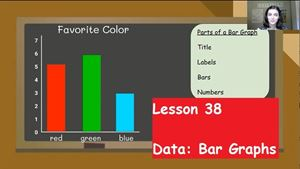Lesson 38 - Data: Bar Graphs, focuses on answering questions about data shown through a bar graph.  This lesson introduces a new term, bar graph, and reviews the term data. The lesson has two exercise documents for students to complete and 2 exercise review videos for students to watch to review the answers and ways to solve the tasks on the exercise.

The lesson video is 16:37.

Exercise 1 contains a bar graph and 6 questions to analyze the data. It should take about 12 minutes to complete.

Exercise 2 contains a bar graph and 6 questions to analyze the data. It should take about 12 minutes to complete.

Please make sure that the student watches the matching exercise review video shortly after finishing the exercise and before moving on.

This lesson most closely aligns with First Grade: CCSS.MATH.CONTENT.1.MD.C.4 “Organize, represent, and interpret data with up to three categories; ask and answer questions about the total number of data points, how many in each category, and how many more or less are in one category than in another.”

This lesson is also related to the following standard.

Second Grade: CCSS.MATH.CONTENT.2.MD.D.10 “Draw a picture graph and a bar graph (with single-unit scale) to represent a data set with up to four categories. Solve simple put-together, take-apart, and compare problems using information presented in a bar graph.”

#### 83 Lesson 38 - Exercise 1 Review 05:46

This video walks students through the answers to the exercise 1 for lesson 38. The video highlights steps and strategies that students should have used when completing the exercise.

The length of the video is 5:46.

#### 84 Lesson 38 - Exercise 2 Review & Test 06:22

This video walks students through the answers to the exercise 2 for lesson 38. The video highlights steps and strategies that students should have used when completing the exercise.

The length of the video is 6:22.

After watching the video, print the MEASUREMENT AND DATA TEST PICTURES.  Then use the pictures to complete the Measurement and Data test.  This test covers counting money, telling time, measuring length, analyzing data and a few review questions.

#### 85 Lesson 39 - Place Value 29:56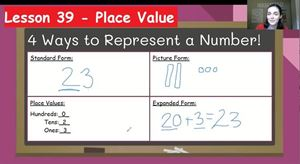Lesson 39 - Place Value, focuses on discussing the value of the digit based on place in a number.  This lesson introduces terms, digit, place value, hundreds, tens, and ones.  This lesson teaches multiple ways to represent numbers, standard form, picture form, and expanded form. The lesson has two exercise documents for students to complete and 2 exercise review videos for students to watch to review the answers and ways to solve the tasks on the exercise.

The lesson video is 29:58.

Exercise 1 contains 8 rows of double digit numbers to represent in various forms. It should take about 16 minutes to complete.

Exercise 2 contains 8 rows of triple digit numbers to represent in various forms. It should take about 16 minutes to complete.

Please make sure that the student watches the matching exercise review video shortly after finishing the exercise and before moving on.

This lesson most closely aligns with Second Grade: CCSS.MATH.CONTENT.2.NBT.A.1 “Understand that the three digits of a three-digit number represent amounts of hundreds, tens, and ones”

This lesson is also related to the following standard.

First Grade: CCSS.MATH.CONTENT.1.NBT.B.2 “Understand that the two digits of a two-digit number represent amounts of tens and ones.”

#### 86 Lesson 39 - Exercise 1 Review 12:10

This video walks students through the answers to the exercise 1 for lesson 39. The video highlights steps and strategies that students should have used when completing the exercise.

The length of the video is 12:10.

#### 87 Lesson 39 - Exercise 2 Review 12:18

This video walks students through the answers to the exercise 2 for lesson 39. The video highlights steps and strategies that students should have used when completing the exercise.

The length of the video is 12:18.

#### 88 Lesson 40 - Comparing Numbers: Within 100 14:34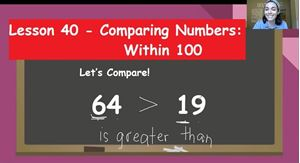Lesson 40 - Comparing Numbers: Within 100, focuses on comparing double digit numbers based on place value.  This lesson introduces terms, greater than, less than, and equal to.  This lesson teaches how to look at place values to compare.  The lesson has one exercise document for students to complete and 1 exercise review video for students to watch to review the answers and ways to solve the tasks on the exercise.

The lesson video is 14:36.

The exercise 5 tasks to compare with words, 8 tasks to compare with the symbol, and 1 sentence to write about which number is greater. It should take about 15 minutes to complete.

Please make sure that the student watches the matching exercise review video shortly after finishing the exercise and before moving on.

This lesson most closely aligns with First Grade: CCSS.MATH.CONTENT.1.NBT.B.3 “Compare two two-digit numbers based on meanings of the tens and ones digits, recording the results of comparisons with the symbols >, =, and <.”

#### 89 Lesson 40 - Exercise Review 09:22

This video walks students through the answers to the exercise for lesson 40. The video highlights steps and strategies that students should have used when completing the exercise.

The length of the video is 9:22.

#### 90 Lesson 41 - Comparing Numbers: Within 1000 14:18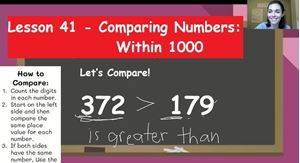Lesson 41 - Comparing Numbers: Within 1000, focuses on comparing triple digit numbers based on place value.  This lesson review terms, greater than, less than, and equal to.  This lesson teaches how to look at place values to compare. The lesson has one exercise document for students to complete and 1 exercise review video for students to watch to review the answers and ways to solve the tasks on the exercise.

The lesson video is 14:20.

The exercise 5 tasks to compare with words, 8 tasks to compare with the symbol, and 1 sentence to write about which number is greater. It should take about 15 minutes to complete.

Please make sure that the student watches the matching exercise review video shortly after finishing the exercise and before moving on.

This lesson most closely aligns with Second Grade: CCSS.MATH.CONTENT.2.NBT.A.4 “Compare two three-digit numbers based on meanings of the hundreds, tens, and ones digits, using >, =, and < symbols to record the results of comparisons.”

#### 91 Lesson 41 - Exercise Review 07:45

This video walks students through the answers to the exercise for lesson 41. The video highlights steps and strategies that students should have used when completing the exercise.

The length of the video is 7:45.

#### 92 Lesson 42 - Ten More, Ten Less 10:46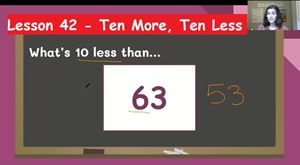Lesson 42 - Ten More, Ten Less, focuses on using place value and counting patterns to mentally tell 10 more or 10 less than a given number.  This lesson introduces the term mental math.  Practice with 10 more and 10 less will help with a later strategy used with double digit addition and subtraction. The lesson has two exercise documents for students to complete and 2 exercise review videos for students to watch to review the answers and ways to solve the tasks on the exercise.

The lesson video is 10:48.

Exercise 1 contains 20 problems about mentally figuring out 10 more or 10 less. It should take about 12 minutes to complete.

Exercise 2 contains 20 problems about mentally figuring out 10 more or 10 less. It should take about 12 minutes to complete.

Please make sure that the student watches the matching exercise review video shortly after finishing the exercise and before moving on.

This lesson most closely aligns with First Grade: CCSS.MATH.CONTENT.1.NBT.C.5 “Given a two-digit number, mentally find 10 more or 10 less than the number, without having to count; explain the reasoning used.”

This lesson is also related to the following standard.

Second Grade: CCSS.MATH.CONTENT.2.NBT.B.8 “Mentally add 10 or 100 to a given number 100-900, and mentally subtract 10 or 100 from a given number 100-900.”

#### 93 Lesson 42 - Exercise 1 Review 10:09

This video walks students through the answers to the exercise 1 for lesson 42. The video highlights steps and strategies that students should have used when completing the exercise.

The length of the video is 10:09.

#### 94 Lesson 42 - Exercise 2 Review & Quiz 09:13

This video walks students through the answers to the exercise 2 for lesson 42. The video highlights steps and strategies that students should have used when completing the exercise.

The length of the video is 9:13.

After watching the video, take the Place Value Quiz.  This quiz covers place value, comparing numbers, and 10 more or 10 less.

#### 95 Lesson 43 - Double Digit Addition: Picture Strategy 18:21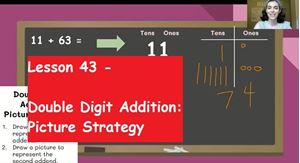Lesson 43 - Double Digit Addition: Picture Strategy, focuses on using pictures of place value blocks to help add double digit numbers.  This lesson reviews important addition terminology. The lesson has two exercise documents for students to complete and 2 exercise review videos for students to watch to review the answers and ways to solve the tasks on the exercise.

The lesson video is 18:23.

Exercise 1 contains 8 problems to solve with the picture strategy. It should take about 15 minutes to complete.

Exercise 2 contains 8 problems to solve with the picture strategy. It should take about 15 minutes to complete.

Please make sure that the student watches the matching exercise review video shortly after finishing the exercise and before moving on.

This lesson most closely aligns with First Grade: CCSS.MATH.CONTENT.1.NBT.C.4 “Add within 100, including adding a two-digit number and a one-digit number, and adding a two-digit number and a multiple of 10, using concrete models or drawings and strategies based on place value, properties of operations, and/or the relationship between addition and subtraction; relate the strategy to a written method and explain the reasoning used. Understand that in adding two-digit numbers, one adds tens and tens, ones and ones; and sometimes it is necessary to compose a ten.”

This lesson is also related to the following standard.

Second Grade: CCSS.MATH.CONTENT.2.NBT.B.5 “Fluently add and subtract within 100 using strategies based on place value, properties of operations, and/or the relationship between addition and subtraction.”

#### 96 Lesson 43 - Exercise 1 Review 09:44

This video walks students through the answers to the exercise 1 for lesson 43. The video highlights steps and strategies that students should have used when completing the exercise.

The length of the video is 9:44.

#### 97 Lesson 43 - Exercise 2 Review 08:50

This video walks students through the answers to the exercise 2 for lesson 43. The video highlights steps and strategies that students should have used when completing the exercise.

The length of the video is 8:50.

#### 98 Lesson 44 - Double Digit Addition: Number Line Strategy 24:09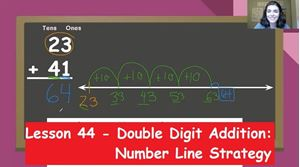Lesson 44 - Double Digit Addition: Number Line Strategy, focuses on using tens and ones jumps on an open number line to add double digit numbers.  This lesson reviews important addition terminology. To complete this activity students must be able to mentally add ten more independently. The lesson has two exercise documents for students to complete and 2 exercise review videos for students to watch to review the answers and ways to solve the tasks on the exercise.

The lesson video is 24:11.

Exercise 1 contains 5 problems to solve with the number line strategy. It should take about 15 minutes to complete.

Exercise 2 contains 5 problems to solve with the number line strategy. It should take about 15 minutes to complete.

Please make sure that the student watches the matching exercise review video shortly after finishing the exercise and before moving on.

This lesson most closely aligns with First Grade: CCSS.MATH.CONTENT.1.NBT.C.4 “Add within 100, including adding a two-digit number and a one-digit number, and adding a two-digit number and a multiple of 10, using concrete models or drawings and strategies based on place value, properties of operations, and/or the relationship between addition and subtraction; relate the strategy to a written method and explain the reasoning used. Understand that in adding two-digit numbers, one adds tens and tens, ones and ones; and sometimes it is necessary to compose a ten.”

This lesson is also related to the following standard.

Second Grade: CCSS.MATH.CONTENT.2.NBT.B.5 “Fluently add and subtract within 100 using strategies based on place value, properties of operations, and/or the relationship between addition and subtraction.”

#### 99 Lesson 44 - Exercise 1 Review 10:03

This video walks students through the answers to the exercise 1 for lesson 44. The video highlights steps and strategies that students should have used when completing the exercise.

The length of the video is 10:03.

#### 100 Lesson 44 - Exercise 2 Review 09:52

This video walks students through the answers to the exercise 2 for lesson 44. The video highlights steps and strategies that students should have used when completing the exercise.

The length of the video is 9:52.

#### 101 Lesson 45 - Double Digit Subtraction: Picture Strategy 15:03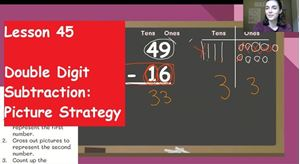Lesson 45 - Double Digit Subtraction: Picture Strategy, focuses on using pictures of place value blocks to subtract double digit numbers.  This lesson reviews important subtraction terminology.  The lesson has two exercise documents for students to complete and 2 exercise review videos for students to watch to review the answers and ways to solve the tasks on the exercise.

The lesson video is 15:05.

Exercise 1 contains 8 problems to solve with the picture strategy. It should take about 15 minutes to complete.

Exercise 2 contains 8 problems to solve with the picture strategy. It should take about 15 minutes to complete.

Please make sure that the student watches the matching exercise review video shortly after finishing the exercise and before moving on.

This lesson most closely aligns with First Grade: CCSS.MATH.CONTENT.1.NBT.C.4 “Add within 100, including adding a two-digit number and a one-digit number, and adding a two-digit number and a multiple of 10, using concrete models or drawings and strategies based on place value, properties of operations, and/or the relationship between addition and subtraction; relate the strategy to a written method and explain the reasoning used. Understand that in adding two-digit numbers, one adds tens and tens, ones and ones; and sometimes it is necessary to compose a ten.”

This lesson is also related to the following standard.

Second Grade: CCSS.MATH.CONTENT.2.NBT.B.5 “Fluently add and subtract within 100 using strategies based on place value, properties of operations, and/or the relationship between addition and subtraction.”

#### 102 Lesson 45 - Exercise 1 Review 08:35

This video walks students through the answers to the exercise 1 for lesson 45. The video highlights steps and strategies that students should have used when completing the exercise.

The length of the video is 8:35.

#### 103 Lesson 45 - Exercise 2 Review 08:27

This video walks students through the answers to the exercise 2 for lesson 45. The video highlights steps and strategies that students should have used when completing the exercise.

The length of the video is 8:27.

#### 104 Lesson 46 - Double Digit Subtraction: Number Line Strategy 17:05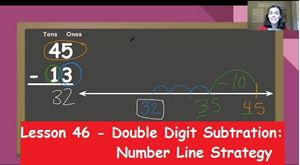Lesson 46 - Double Digit Subtraction: Number Line Strategy, focuses on using tens and ones jumps on a number line to subtract double digit numbers.  This lesson reviews important subtraction terminology. To complete this activity students need to be able to do mental math for 10 less independently.  The lesson has two exercise documents for students to complete and 2 exercise review videos for students to watch to review the answers and ways to solve the tasks on the exercise.

The lesson video is 17:07.

Exercise 1 contains 5 problems to solve with the number line strategy. It should take about 15 minutes to complete.

Exercise 2 contains 5 problems to solve with the number line strategy. It should take about 15 minutes to complete.

Please make sure that the student watches the matching exercise review video shortly after finishing the exercise and before moving on.

This lesson most closely aligns with First Grade: CCSS.MATH.CONTENT.1.NBT.C.4 “Add within 100, including adding a two-digit number and a one-digit number, and adding a two-digit number and a multiple of 10, using concrete models or drawings and strategies based on place value, properties of operations, and/or the relationship between addition and subtraction; relate the strategy to a written method and explain the reasoning used. Understand that in adding two-digit numbers, one adds tens and tens, ones and ones; and sometimes it is necessary to compose a ten.”

This lesson is also related to the following standard.

Second Grade: CCSS.MATH.CONTENT.2.NBT.B.5 “Fluently add and subtract within 100 using strategies based on place value, properties of operations, and/or the relationship between addition and subtraction.”

#### 105 Lesson 46 - Exercise 1 Review 08:19

This video walks students through the answers to the exercise 1 for lesson 46. The video highlights steps and strategies that students should have used when completing the exercise.

The length of the video is 8:19.

#### 106 Lesson 46 - Exercise 2 Review 09:54

This video walks students through the answers to the exercise 2 for lesson 46. The video highlights steps and strategies that students should have used when completing the exercise.

The length of the video is 9:54.

#### 107 Lesson 47 - Double Digit Mixed Review 13:58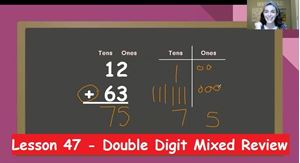Lesson 47 - Double Digit Mixed Review, focuses on paying attention to the operation in the math problems and using both strategies to solve.  This lesson reviews important addition and subtraction terminology. To complete this activity students need to be able to do mental math for 10 more and 10 less independently.  The lesson has two exercise documents for students to complete and 2 exercise review videos for students to watch to review the answers and ways to solve the tasks on the exercise.

The lesson video is 13:59.

Exercise 1 contains 6 problems to solve with the picture strategy and 2 problems to solve with number line strategy. It should take about 15 minutes to complete.

Exercise 2 contains 6 problems to solve with the picture strategy and 2 problems to solve with number line strategy. It should take about 15 minutes to complete.

Please make sure that the student watches the matching exercise review video shortly after finishing the exercise and before moving on.

This lesson most closely aligns with First Grade: CCSS.MATH.CONTENT.1.NBT.C.4 “Add within 100, including adding a two-digit number and a one-digit number, and adding a two-digit number and a multiple of 10, using concrete models or drawings and strategies based on place value, properties of operations, and/or the relationship between addition and subtraction; relate the strategy to a written method and explain the reasoning used. Understand that in adding two-digit numbers, one adds tens and tens, ones and ones; and sometimes it is necessary to compose a ten.”

This lesson is also related to the following standard.

Second Grade: CCSS.MATH.CONTENT.2.NBT.B.5 “Fluently add and subtract within 100 using strategies based on place value, properties of operations, and/or the relationship between addition and subtraction.”

#### 108 Lesson 47 - Exercise 1 Review 10:07

This video walks students through the answers to the exercise 1 for lesson 47. The video highlights steps and strategies that students should have used when completing the exercise.

The length of the video is 10:07.

#### 109 Lesson 47 - Exercise 2 Review & Test 09:55

This video walks students through the answers to the exercise 2 for lesson 47. The video highlights steps and strategies that students should have used when completing the exercise.

The length of the video is 9:55.

After this video, students will take a test.  Please print the document called PLACE VALUE AND DOUBLE DIGIT TEST PICTURES before beginning the test.  This test will focus on place value, comparing numbers, along with addition and subtraction for double digit numbers.  The test will also include a few review questions as well.

#### 110 Lesson 48 - Triple Digit Addition 14:49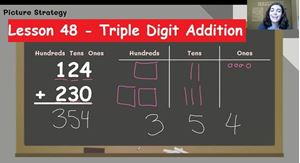Lesson 48 - Triple Digit Addition, focuses on solving triple digit addition problems.  This lesson reviews important addition terminology and place value. This lesson provides an overview of 3 different strategies to choose from when adding three digit numbers. The lesson has two exercise documents for students to complete and 2 exercise review videos for students to watch to review the answers and ways to solve the tasks on the exercise.

The lesson video is 14:51.

Exercise 1 contains 5 problems to solve and it should take about 10 minutes to complete.

Exercise 2 contains 5 problems to solve and it should take about 10 minutes to complete.

Please make sure that the student watches the matching exercise review video shortly after finishing the exercise and before moving on.

This lesson most closely aligns with Second Grade: CCSS.MATH.CONTENT.2.NBT.B.7 “Add and subtract within 1000, using concrete models or drawings and strategies based on place value, properties of operations, and/or the relationship between addition and subtraction; relate the strategy to a written method. Understand that in adding or subtracting three-digit numbers, one adds or subtracts hundreds and hundreds, tens and tens, ones and ones; and sometimes it is necessary to compose or decompose tens or hundreds.”

#### 111 Lesson 48 - Exercise 1 Review 05:33

This video walks students through the answers to the exercise 1 for lesson 48. The video highlights steps and strategies that students should have used when completing the exercise.

The length of the video is 5:33.

#### 112 Lesson 48 - Exercise 2 Review 04:33

This video walks students through the answers to the exercise 2 for lesson 48. The video highlights steps and strategies that students should have used when completing the exercise.

The length of the video is 4:33.

#### 113 Lesson 49 - Triple Digit Subtraction 16:23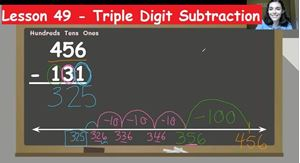Lesson 49 - Triple Digit Subtraction, focuses on solving triple digit subtraction problems.  This lesson reviews important subtraction terminology and place value. This lesson provides an overview of 3 different strategies to choose from when subtracting three digit numbers. The lesson has two exercise documents for students to complete and 2 exercise review videos for students to watch to review the answers and ways to solve the tasks on the exercise.

The lesson video is 16:25.

Exercise 1 contains 5 problems to solve and it should take about 10 minutes to complete.

Exercise 2 contains 5 problems to solve and it should take about 10 minutes to complete.

Please make sure that the student watches the matching exercise review video shortly after finishing the exercise and before moving on.

This lesson most closely aligns with Second Grade: CCSS.MATH.CONTENT.2.NBT.B.7 “Add and subtract within 1000, using concrete models or drawings and strategies based on place value, properties of operations, and/or the relationship between addition and subtraction; relate the strategy to a written method. Understand that in adding or subtracting three-digit numbers, one adds or subtracts hundreds and hundreds, tens and tens, ones and ones; and sometimes it is necessary to compose or decompose tens or hundreds.”

#### 114 Lesson 49 - Exercise 1 Review 06:20

This video walks students through the answers to the exercise 1 for lesson 49. The video highlights steps and strategies that students should have used when completing the exercise.

The length of the video is 6:20.

#### 115 Lesson 49 - Exercise 2 Review 06:50

This video walks students through the answers to the exercise 2 for lesson 49. The video highlights steps and strategies that students should have used when completing the exercise.

The length of the video is 6:50.

#### 116 Lesson 50 - Addition with Regrouping 22:49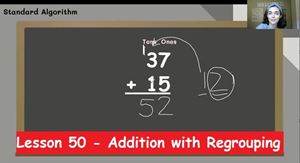Lesson 50 - Addition with Regrouping, focuses on solving addition problems that involve regrouping one into a bundle of ten.  This lesson reviews important addition terminology and place value. This lesson provides an overview of 2 different strategies to choose from when adding a math problem with regrouping. The lesson has two exercise documents for students to complete and 2 exercise review videos for students to watch to review the answers and ways to solve the tasks on the exercise.

The lesson video is 22:51.

Exercise 1 contains 8 problems to solve and it should take about 16 minutes to complete.

Exercise 2 contains 8 problems to solve and it should take about 16 minutes to complete.

Please make sure that the student watches the matching exercise review video shortly after finishing the exercise and before moving on.

This lesson most closely aligns with First Grade: CCSS.MATH.CONTENT.1.NBT.C.4 “Add within 100, including adding a two-digit number and a one-digit number, and adding a two-digit number and a multiple of 10, using concrete models or drawings and strategies based on place value, properties of operations, and/or the relationship between addition and subtraction; relate the strategy to a written method and explain the reasoning used. Understand that in adding two-digit numbers, one adds tens and tens, ones and ones; and sometimes it is necessary to compose a ten.”

This lesson is also related to the following standard.

Second Grade:CCSS.MATH.CONTENT.2.NBT.B.7 “Add and subtract within 1000, using concrete models or drawings and strategies based on place value, properties of operations, and/or the relationship between addition and subtraction; relate the strategy to a written method. Understand that in adding or subtracting three-digit numbers, one adds or subtracts hundreds and hundreds, tens and tens, ones and ones; and sometimes it is necessary to compose or decompose tens or hundreds.”

#### 117 Lesson 50 - Exercise 1 Regrouping 17:22

This video walks students through the answers to the exercise 1 for lesson 50. The video highlights steps and strategies that students should have used when completing the exercise.

The length of the video is 17:22.

#### 118 Lesson 50 - Exercise 2 Regrouping 09:00

This video walks students through the answers to the exercise 2 for lesson 50. The video highlights steps and strategies that students should have used when completing the exercise.

The length of the video is 9:00.

#### 119 Lesson 51 - Subtraction with Regrouping 20:39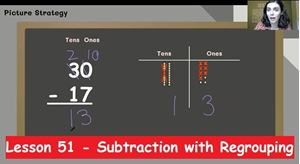Lesson 51 - Subtraction with Regrouping, focuses on solving subtraction problems that involve regrouping a ten to create more ones.  This lesson reviews important subtraction terminology and place value. This lesson provides an overview of 2 different strategies to choose from when subtracting a math problem with regrouping. The lesson has two exercise documents for students to complete and 2 exercise review videos for students to watch to review the answers and ways to solve the tasks on the exercise.

The lesson video is 20:40.

Exercise 1 contains 8 problems to solve and it should take about 16 minutes to complete.

Exercise 2 contains 8 problems to solve and it should take about 16 minutes to complete.

Please make sure that the student watches the matching exercise review video shortly after finishing the exercise and before moving on.

This lesson most closely aligns with Second Grade: CCSS.MATH.CONTENT.2.NBT.B.7 “Add and subtract within 1000, using concrete models or drawings and strategies based on place value, properties of operations, and/or the relationship between addition and subtraction; relate the strategy to a written method. Understand that in adding or subtracting three-digit numbers, one adds or subtracts hundreds and hundreds, tens and tens, ones and ones; and sometimes it is necessary to compose or decompose tens or hundreds.”

#### 120 Lesson 51 - Exercise 1 Review 15:21

This video walks students through the answers to the exercise 1 for lesson 51. The video highlights steps and strategies that students should have used when completing the exercise.

The length of the video is 15:21.

#### 121 Lesson 51 - Exercise 2 Review 07:28

This video walks students through the answers to the exercise 2 for lesson 51. The video highlights steps and strategies that students should have used when completing the exercise.

The length of the video is 7:28.

#### 122 Lesson 52 - Double Digit Mixed Review with Regrouping 14:31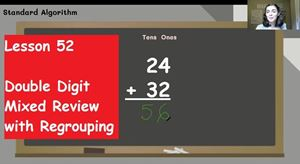Lesson 52 - Double Digit Mixed Review with Regrouping, focuses on solving double digit addition and subtraction problems and determining if regrouping needs to be done.  This lesson reviews important  addition and subtraction terminology and place value. This lesson reminds students of all topics discussed with double digit addition and subtraction. The lesson has two exercise documents for students to complete and 2 exercise review videos for students to watch to review the answers and ways to solve the tasks on the exercise.

The lesson video is 14:33.

Exercise 1 contains 8 problems to solve and it should take about 16 minutes to complete.

Exercise 2 contains 8 problems to solve and it should take about 16 minutes to complete.

Please make sure that the student watches the matching exercise review video shortly after finishing the exercise and before moving on.

This lesson most closely aligns with Second Grade: CCSS.MATH.CONTENT.2.NBT.B.7 “Add and subtract within 1000, using concrete models or drawings and strategies based on place value, properties of operations, and/or the relationship between addition and subtraction; relate the strategy to a written method. Understand that in adding or subtracting three-digit numbers, one adds or subtracts hundreds and hundreds, tens and tens, ones and ones; and sometimes it is necessary to compose or decompose tens or hundreds.”

#### 123 Lesson 52 - Exercise 1 Review 08:09

This video walks students through the answers to the exercise 1 for lesson 52. The video highlights steps and strategies that students should have used when completing the exercise.

The length of the video is 8:09.

#### 124 Lesson 52 - Exercise 2 Review 08:12

This video walks students through the answers to the exercise 2 for lesson 52. The video highlights steps and strategies that students should have used when completing the exercise.

The length of the video is 8:12.

#### 125 Lesson 53 - Arrays 25:31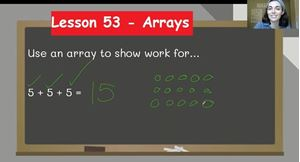Lesson 53 - Arrays, focuses on creating arrays to match equations for repeated addition.  This lesson introduces the terms arrays and repeated addition. This lesson reminds students of key addition terminology. The lesson has two exercise documents for students to complete and 2 exercise review videos for students to watch to review the answers and ways to solve the tasks on the exercise.

The lesson video is 25:33.

Exercise 1 contains 4 equations to solve and 1 story problem to complete.  It should take about 10 minutes to complete.

Exercise 2 contains 4 equations to solve and 1 story problem to complete.  It should take about 10 minutes to complete.

Please make sure that the student watches the matching exercise review video shortly after finishing the exercise and before moving on.

This lesson most closely aligns with Second Grade: CCSS.MATH.CONTENT.2.OA.C.4 “Use addition to find the total number of objects arranged in rectangular arrays with up to 5 rows and up to 5 columns; write an equation to express the total as a sum of equal addends.”

#### 126 Lesson 53 - Exercise 1 Review 06:50

This video walks students through the answers to the exercise 1 for lesson 53. The video highlights steps and strategies that students should have used when completing the exercise.

The length of the video is 6:50.

#### 127 Lesson 53 - Exercise 2 Review & Quiz 06:28

This video walks students through the answers to the exercise 2 for lesson 53. The video highlights steps and strategies that students should have used when completing the exercise.

The length of the video is 6:28.

After the video there is a Quiz that covers all the content from the 2nd Grade Enrichment Section.  It covers triple digit addition, triple digit subtraction, regrouping, arrays.

Course Description

Early Elementary Math. This complete math course covers all of the major points taught across NC and closely aligns with standards taught across the USA. This course is ideal for first grade students; however, kindergartens with strong number sense will enjoy the challenge and 2nd graders needing a slower paced math course would benefit as well! This course reviews some kindergarten to ensure that students have the prerequisite knowledge for the 1st grade lessons.  Many lessons also introduce 2nd grade content to ensure that students will be fully prepared for the next grade! The course consists of 53 content rich video-lessons, 67 teacher created exercise worksheets (and their answer keys), 67 exercise review videos, 9 quizzes, 4 tests and additional resources which parents will have immediate access to upon purchasing this course.

In this course students are encouraged to have a paper and pencil during the video lessons to work along with the teacher after viewing example questions first.  Students are encouraged to not just think about the next step, but why we need to take the next step.  We are trying to build a mathematical foundation based on understanding instead of memorization!

Course Goals

Upon course completion, students will have an understanding of important math concepts to ensure that they are prepared to move on to increasingly challenging 2nd and 3rd grade math skills.

Target Audience

This video-course is primarily intended for Grade 1 students (typically ages 6+).  Kindergarten students with strong number sense, who are in need of a challenge will enjoy this course.  Grade 2 students who may need to review previously taught concepts while easing into the 2nd grade skills will also benefit from this course. The difficulty level of this course is easy to medium for the first 36 lessons.  The course difficulty goes from medium-hard  to hard during the last 17 lessons.  The final unit is entirely composed of the most challenging 2nd grade topics that will be discussed.

Course Requirements

Students taking this course are expected to be independent with many Kindergarten math concepts.  Students must be able to do addition and subtraction within 5, compare numbers from 0 to 10, and have some familiarity with counting to 100.

Course Includes

• Approximately 14.5 hours of lesson videos
• 53 Video-lessons
• 67 Practice exercises
• 67 Worksheet review videos (I go over each activity and explain each solution one by one)
• 9 Quizzes
• 4 Tests
• Answer "keys" to all practice worksheets
• Resource Videos for Counting and Skip Counting Practice
• Resource 100 Chart and 150 Chart

Course Topics

• Counting Objects in a Set
• Teens
• Patterns Counting to 100
• Patterns Counting to 150
• Odd or Even
• Basic Subtraction Strategies
• Story Problems with Addition and Subtraction
• True or False Math
• Unknowns Parts
• Unknowns Parts Story Problems
• 2D shapes
• Composite shapes
• 3D shapes
• 2D vs 3D shapes
• Fractions (half, thirds, fourths)
• Coins
• Telling Time to the Hour
• Telling Time to Half Hour
• Time to Increments of 5
• Comparing Lengths
• Measuring with NonStandard Units
• Measuring with a Ruler
• Bar graph and analysis
• Pictograph and analysis
• Place Value
• Comparing Numbers within 100
• Comparing Numbers within 1000
• Ten More, Ten Less Mental Math
• Double digit subtraction
• Triple digit subtraction
• Regrouping subtraction
• Arrays

• Teacher: Caitlin
• Areas of expertise: Elementary Education
• Education: B.A. in Elementary Education with a concentration in Health and Science from UNCW
• Interests: Reading mystery novels, Testing out new recipes with my husband, Playing Animal Crossing: New Horizons
• Skills: Teaching early elementary grades phonics, math, reading comprehension, science, and social studies.
• Associations: NC Elementary School Teacher
• Issues I care about: Caring for the Environment and Equity in Education

I am passionate about education! I have been a 1st grade teacher in NC for 5 years. Growing up I loved learning about math, and now as an adult I love teaching it! I also enjoy phonics, science, and history. In students early years at school there is a lot of important foundation to create. I enjoy helping students gain a true understanding of skills, to ensure that they will continue to remember what they learned and be successful as they go through all their school years!

#### Test Preparation Document

Test Preparation Document

#### Digital Quizzes and Tests Answer Keys Document

Digital Quizzes and Tests Answer Keys Document

#### 100 Chart

A chart of numbers from 1 to 100. Students can look at this to help practice counting before they are ready to count from memory.

#### Blank 100 Chart

An empty chart with 100 boxes. Students can practice writing their numbers from 1 to 100 in this blank chart.

#### 150 Chart

A chart of numbers from 1 to 150. Students can look at this to help practice counting before they are ready to count from memory.

#### Blank 150 Chart

An empty chart with 150 boxes. Students can practice writing their numbers from 1 to 150 in this blank chart.

#### Number Lines to 15

This page has 5 blank number lines from 1 to 15.  Students can use these number line to help add and subtract within 15.

#### Counting to 100

This video is good for students who need extra practice counting to 100.

#### Counting to 150

This video is good for students who need extra practice counting to 150.

#### Skip Counting by 10

This video is good for students who need extra practice skip counting by 10 up to 100.

#### Skip Counting by 5

This video is good for students who need extra practice skip counting by 5 up to 100.

#### Skip Counting by 2

This video is good for students who need extra practice skip counting by 2 up to 100.

#### Lesson 1 - ANSWER KEY

This is the answer key for the Lesson 1 exercise.

#### Lesson 2 - ANSWER KEY

This is the answer key to the Teens Exercise for Lesson 2.

#### Lesson 3 - ANSWER KEY

This is the answer key to the Patterns Counting to 100 Exercise for Lesson 3

#### Lesson 4 - ANSWER KEY

This is the answer key to the exercise for Lesson 4.

#### Lesson 6 - ANSWER KEY

This is the answer key to the exercise for Lesson 6.

#### Lesson 7 - ANSWER KEY

This is the answer key for Lesson 7.

#### Lesson 8 - ANSWER KEY

This is the answer key for the exercise for Lesson 8.

#### Lesson 9 - ANSWER KEY

This is the answer key for the Lesson 9 exercise.

#### Lesson 10 - ANSWER KEY

This is the answer key that matches with the Lesson 10 exercise.

#### Lesson 11 - ANSWER KEY

This is the answer key for the Lesson 11 exercise.

#### Lesson 12 - ANSWER KEY

This is the answer key that matches with the Lesson 12 exercise.

#### Lesson 13 - ANSWER KEY

This is the answer key that matches with the Lesson 13 exercise.

#### Lesson 14 - ANSWER KEY

This is the answer key for the Lesson 14 exercise.

#### Lesson 15 - ANSWER KEY

This is the answer key for the Lesson 15 exercise.

#### Lesson 16 - ANSWER KEY

This answer key matches with the exercise for Lesson 16.

#### Lesson 17 - ANSWER KEY

This is the answer key for the exercise for Lesson 17.

#### Lesson 18 - ANSWER KEYS

These are the answer keys for both exercise 1 and exercise 2 of Lesson 18.

#### Lesson 19 - ANSWER KEYS

These are the answer keys for the first AND second exercises for Lesson 19.

#### Lesson 20 - ANSWER KEY

This is the answer key that matches with the exercise for Lesson 20.

#### Lesson 21 - ANSWER KEY

This is the answer key that matches with the exercise for Lesson 21.

#### Lesson 22 - ANSWER KEY

This is the answer key that matches with the exercise for Lesson 22.

#### Lesson 23 - ANSWER KEY

This is the answer key that matches with the exercise for Lesson 23

#### Lesson 24 - ANSWER KEY

This is the answer key for the exercise that matches with Lesson 24.

#### Lesson 25 - ANSWER KEY

This is the answer key that matches with the exercise for Lesson 25.

#### Lesson 26 - ANSWER KEYS

These are the answer keys that match with the first exercise and second exercise for lesson 26.

#### Lesson 27 - ANSWER KEY

This is the answer key that matches with the exercise for lesson 27.

#### Lesson 28 - ANSWER KEYS

These are the answer keys that match with the first and second exercises for Lesson 28

#### Lesson 29 - ANSWER KEY

This is the answer key that matches with the exercise for Lesson 29.

#### Lesson 30 - ANSWER KEYS

These are the answer keys for the first exercise and second exercise that match with Lesson 30

#### Lesson 31 - ANSWER KEYS

These are the answer keys that match with the first and second exercises for Lesson 31.

#### Lesson 32 - ANSWER KEY

This is the answer key that matches with the exercise for Lesson 32.

#### Lesson 33 - ANSWER KEY

This is the answer key for the exercise that matches with Lesson 33.

#### Lesson 34 - ANSWER KEY

This is the answer key that matches with the exercise for Lesson 34.

#### Lesson 35 - ANSWER KEY

This is the answer key that matches with the exercise for Lesson 35.

#### Lesson 36 - ANSWER KEY

This is the answer key that matches with the exercise for lesson 36.

#### Lesson 37 - ANSWER KEYS

These are the answer keys to the first and second exercises for lesson 37.

#### Lesson 38 - ANSWER KEYS

These are the answer keys that match with the first and second exercises for Lesson 38.

#### Lesson 39 - ANSWER KEYS

These are the answer key that matches with the first and second exercises that match Lesson 39.

#### Lesson 40 - ANSWER KEY

This is the answer key that matches with the exercise for Lesson 40.

#### Lesson 41 - ANSWER KEY

This is the answer key that matches with the exercise for lesson 41.

#### Lesson 42 - ANSWER KEYS

These are the answer keys that match with the first and second exercises for Lesson 42.

#### Lesson 43 - ANSWER KEYS

These are the answer keys that match with the first and second exercises for Lesson 43.

#### Lesson 44 - ANSWER KEYS

These are the answer keys that match with the first and second exercises for Lesson 44.

#### Lesson 45 - ANSWER KEYS

These are the answer keys that match with the first and second exercises for Lesson 45.

#### Lesson 46 - ANSWER KEYS

These the answer keys that match with the first and second exercises for Lesson 46.

#### Lesson 47 - ANSWER KEYS

These are the answer keys that match with the first and second exercises for Lesson 47.

#### Lesson 48 - ANSWER KEYS

These are the answer keys that match with the first and second exercises for Lesson 48.

#### Lesson 49 - ANSWER KEYS

These are the answer keys that match with the first and second exercises for Lesson 49

#### Lesson 50 - ANSWER KEYS

These are the answer keys that match with the first and second exercises for Lesson 50.

#### Lesson 51 - ANSWER KEYS

These are the answer keys that match with the first and second exercises for Lesson 51.

#### Lesson 52 - ANSWER KEYS

These are the answer keys for the first and second exercises that match with Lesson 52.

#### Lesson 53 - ANSWER KEYS

These are the answer keys that match with the first and second exercises for Lesson 53.

This is the answer key to the Counting Quiz. The Counting Quiz is attached to the exercise review video for lesson 5 and should take students about 16 minutes.

This is the answer key to the Addition and Subtraction Quiz. The Addition and Subtraction Quiz is attached to the exercise review video for lesson 13 and should take students about 8 minutes.

#### Story Problems Quiz ANSWER KEY

This is the answer key to the Story Problems Quiz. The Story Problems Quiz is attached to the exercise review video for lesson 16 and should take students about 12 minutes.

#### True or False and Unknow Parts Quiz ANSWER KEY

This is the answer key to the True or False and Unknow Parts Quiz. The True or False and Unknow Parts Quiz is attached to the final exercise review video for lesson 19 and should take students about 10 minutes.

#### Counting and Operations Tests ANSWER KEY

This is the answer key to the Counting and Operations Tests. The Counting and Operations Tests is attached to the exercise review video for lesson 21 and should take students about 35 minutes.

This is the answer key to the Shapes Quiz. The Shapes Quiz is attached to the exercise review video for lesson 25 and should take students about 15 minutes.

This is the answer key to the Geometry Test. The Geometry Test is attached to the exercise review video for lesson 26 and should take students about 35 minutes.

This is the answer key to the Coins Quiz. The Coins Quiz is attached to the exercise review video for lesson 28 and should take students about 16 minutes.

#### Telling Time Quiz ANSWER KEY

This is the answer key to the Telling Time Quiz. The Telling Time Quiz is attached to the exercise review video for lesson 31 and should take students about 16 minutes.

This is the answer key to the Measurement Quiz. The Measurement Quiz is attached to the exercise review video for lesson 36 and should take students about 16 minutes.

#### Measurement and Data Test ANSWER KEY

This is the answer key to the Measurement and Data Test. The Measurement and Data Test is attached to the exercise review video for lesson 38 and should take students about 35 minutes.

#### Place Value Quiz ANSWER KEY

This is the answer key to the Place Value Quiz. The Place Value Quiz is attached to the final exercise review video for lesson 42 and should take students about 20 minutes.

#### Place Value and Double Digit Test ANSWER KEY

This is the answer key to the Place Value and Double Digit Test. The Place Value and Double Digit Test is attached to the final exercise review video for lesson 47 and should take students about 45 minutes.

This is the answer key to the 2nd Grade Enrichment Quiz. The 2nd Grade Enrichment Quiz is attached to the final exercise review video for lesson 53 and should take students about 20 minutes.

Customers who bought this item also bought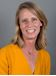Enter the amazing world of asking questions, exploring answers, and sharing observations. Our world and all that surrounds us is full of curious and sometimes bewildering things! Prepare to open your eyes and follow the path of adventure ahead. +
\$199.00 \$93.00Designed for students ages six to nine, Mindfulness for Kids offers an introduction to mindfulness practices and techniques for building awareness, developing resiliency, managing emotions and nurturing compassion. +
\$199.00 \$93.00This course will provide lessons and content based on common skills that students will master within 2nd grade, such as addition, subtraction, measurment of time, and multiplication. +
\$275.00 \$129.00